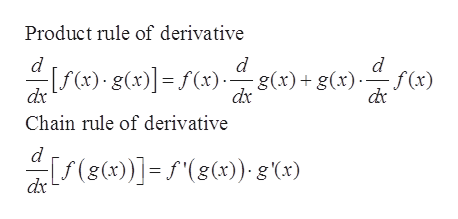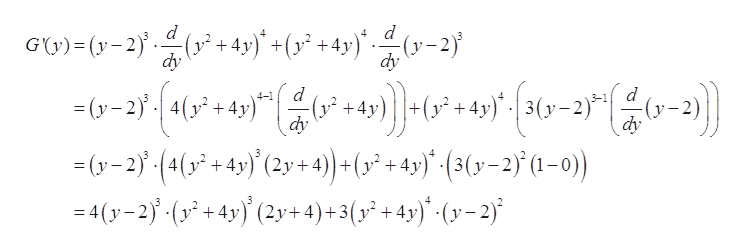# G(y)=(y − 2)3(y2 + 4y)4Find G'(y).Side note: This is the answer I got which is wrong, but the answer has to written in this same format.(y-2)2 (-y2+12y+8)(y2+4y)13

Question
12 views

G(y)=

 (y − 2)3 (y2 + 4y)4

Find G'(y).

Side note: This is the answer I got which is wrong, but the answer has to written in this same format.

 (y-2)2 (-y2+12y+8) (y2+4y)13
check_circle

Step 1

As the function G(y) is not clarified and well formatted.
Therefore, taking the function G(y) = (y – 2)2(y2 + 4y)4.

Step 2

Formula used:help_outlineImage TranscriptioncloseProduct rule of derivative d d d - [f()- g(x)] = f(x)- g(x)+g(x)f(x) dx dx dr Chain rule of derivative d (g(x)] = f"(g(x)- g'(x) dx fullscreen
Step 3

Differentiating G(y) wi...help_outlineImage Transcriptionclosed d GU)-(y-2 4y)+(+4y)-2 dy d d =(y-2 4( +4y dy +4y dy -(y-2(4(+4)(2y+ 4)-(+4y) (3(y-2 (1-0) 4(y2(4(2y+4)+3(? + 4y)" (y=2} fullscreen

### Want to see the full answer?

See Solution

#### Want to see this answer and more?

Solutions are written by subject experts who are available 24/7. Questions are typically answered within 1 hour.*

See Solution
*Response times may vary by subject and question.
Tagged in

### Derivative